# # 剑指 Offer 45. 把数组排成最小的数

## # 一、题目描述

``````输入: [10,2]

``````

``````输入: [3,30,34,5,9]

``````

• `0 < nums.length <= 100`

• 输出结果可能非常大，所以你需要返回一个字符串而不是整数
• 拼接起来的数字可能会有前导 0，最后结果不需要去掉前导 0

## # 二、题目解析

``````输入: [10,2]
``````

• 1、“ 10 ” + “ 2 ” = “ 102 ”
• 2、“ 2 ” + “ 10 ” = “ 210 ”

102 是小于 210 的，也就意味着拼接过程中 10 应该放到 2 的前面。

• 1、“ m ” + “ n ” = “ mn ”
• 2、“ n ” + “ m ” = “ nm ”

• 1、当 mn < nm 时，选取 mn
• 2、当 nm < mn 时，选取 nm

``````输入: [3,30,34,1,9]
``````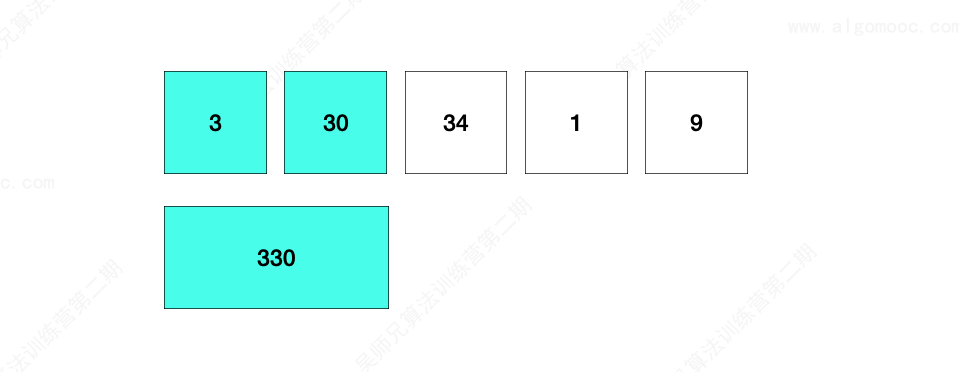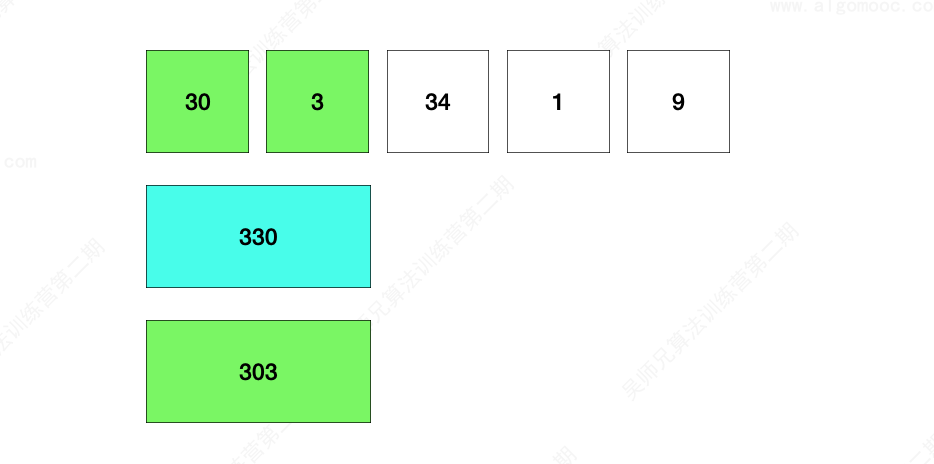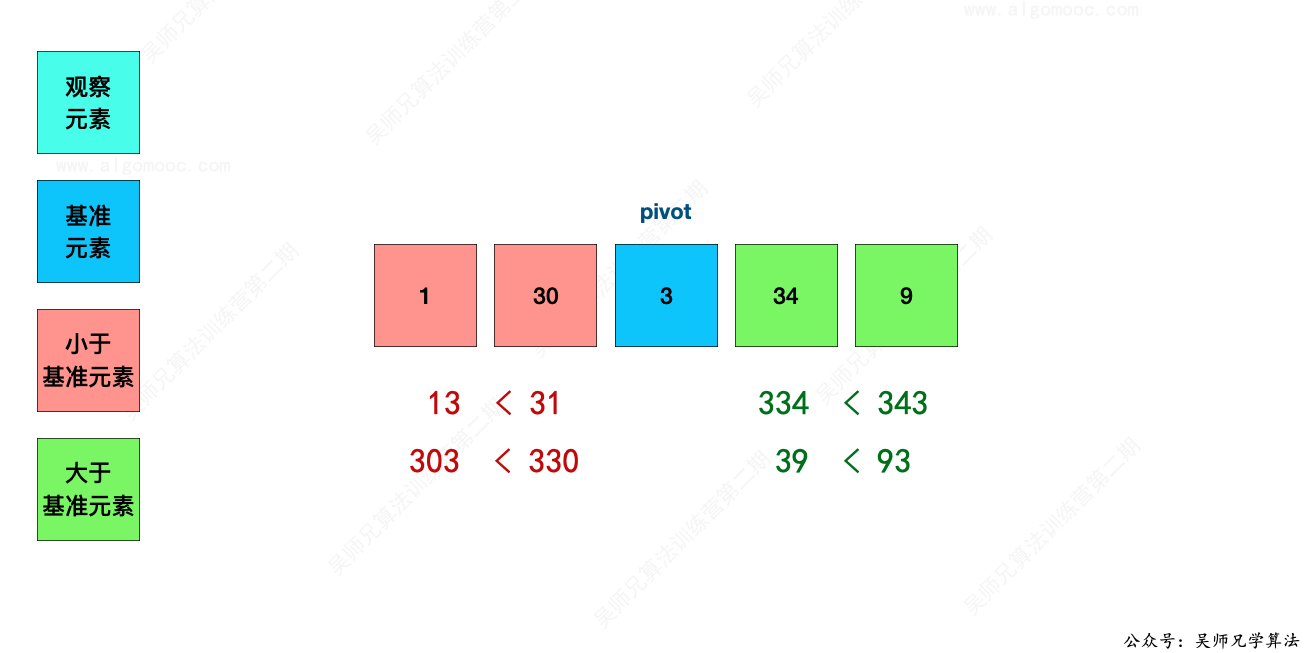1、红色区域的任意数字和蓝色区域的任意数字进行拼接，都是会小于蓝色区域的任意数字和红色区域的任意数字进行拼接。

2、蓝色区域的任意数字和绿色区域的任意数字进行拼接，都是会小于绿色区域的任意数字和蓝色区域的任意数字进行拼接。

1、题目说明输出结果可能非常大，需要返回一个字符串而不是整数，那么第一步就先把整型数组转换为字符串数组。

2、接下来开始对这个字符串数组进行排序操作。

3、选取第一个元素作为基准值，以这个基准值作为基础，先把字符串数组划分为三个部分：

• 左边的部分和基准值进行拼接的字符串会小于基准值和左边的部分进行拼接的字符串
• 基准值和右边的部分进行拼接的字符串会小于右边的部分和基准值进行拼接的字符串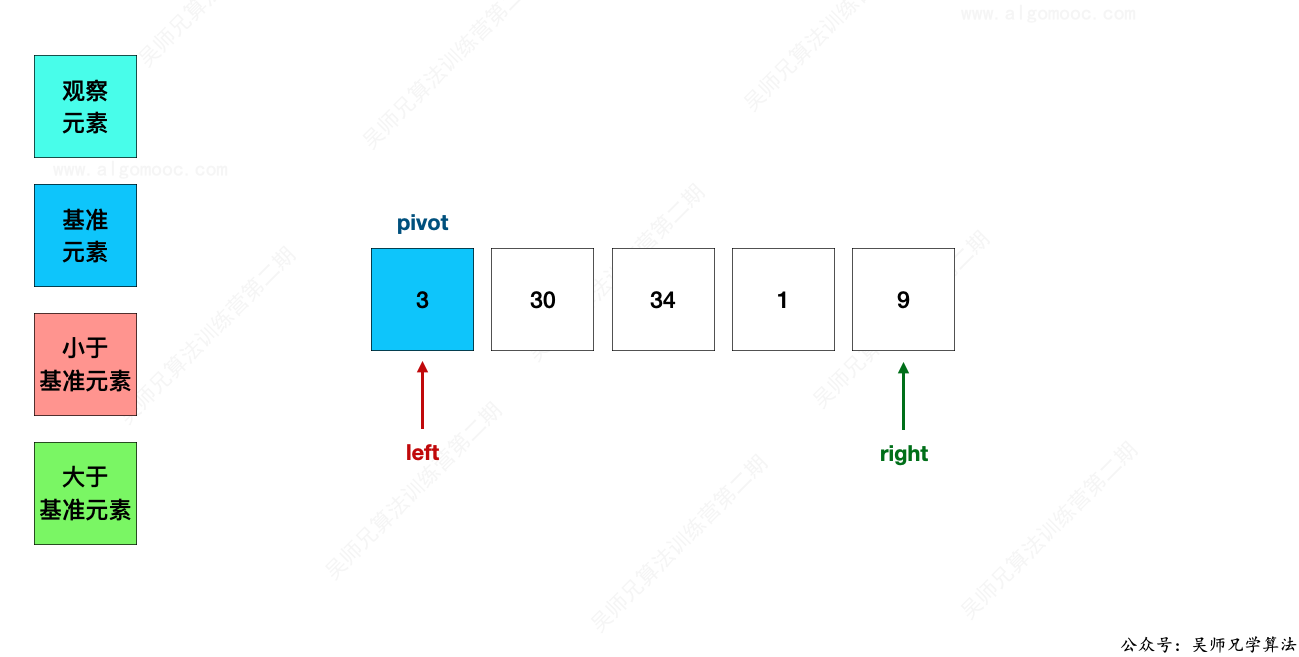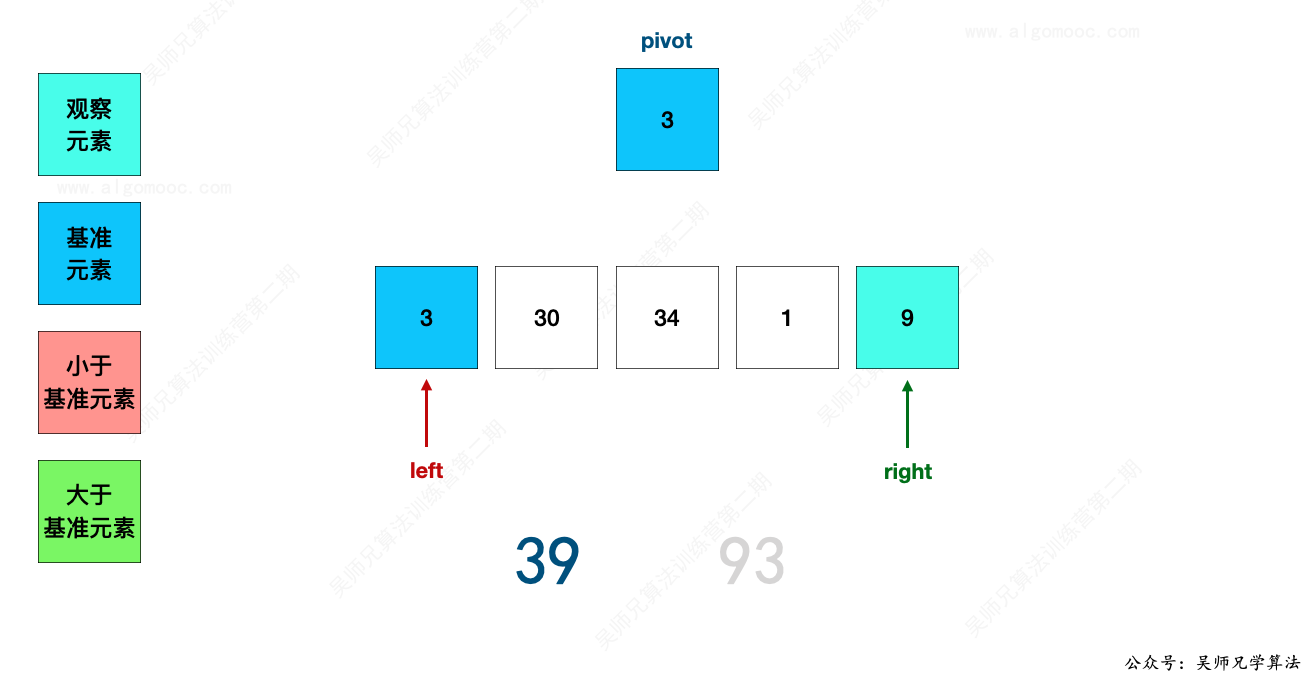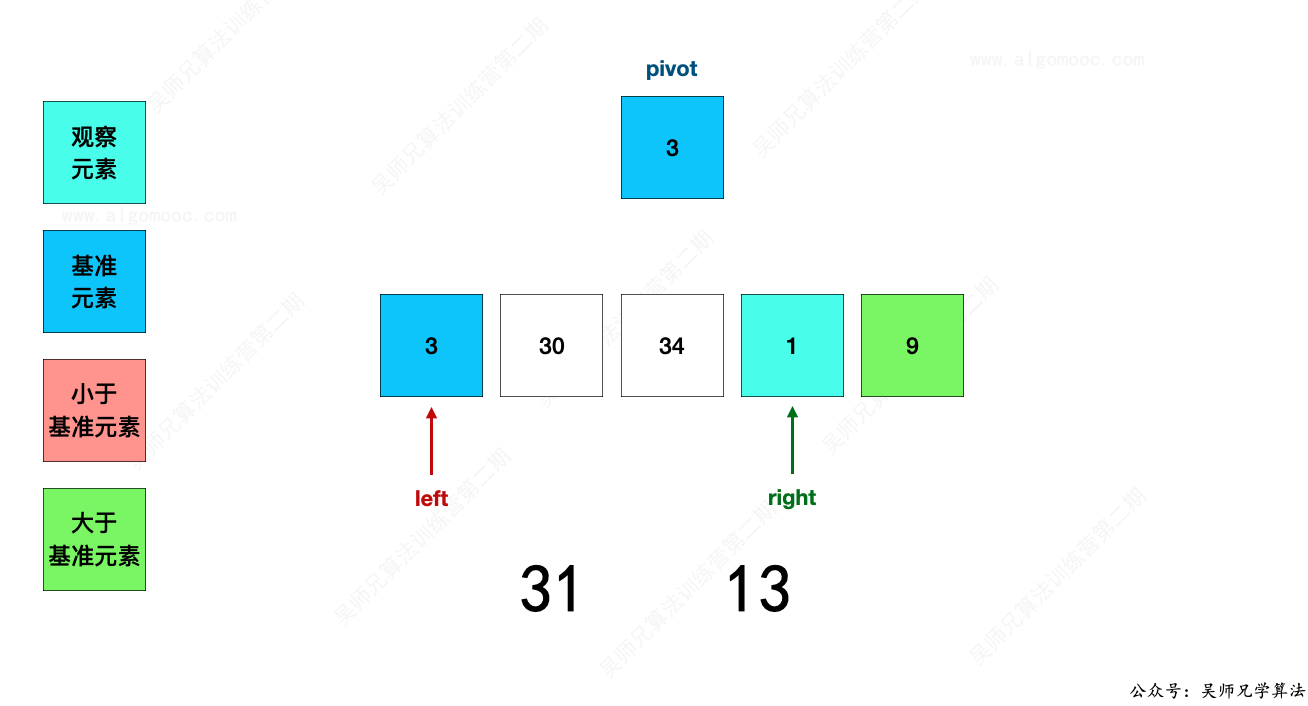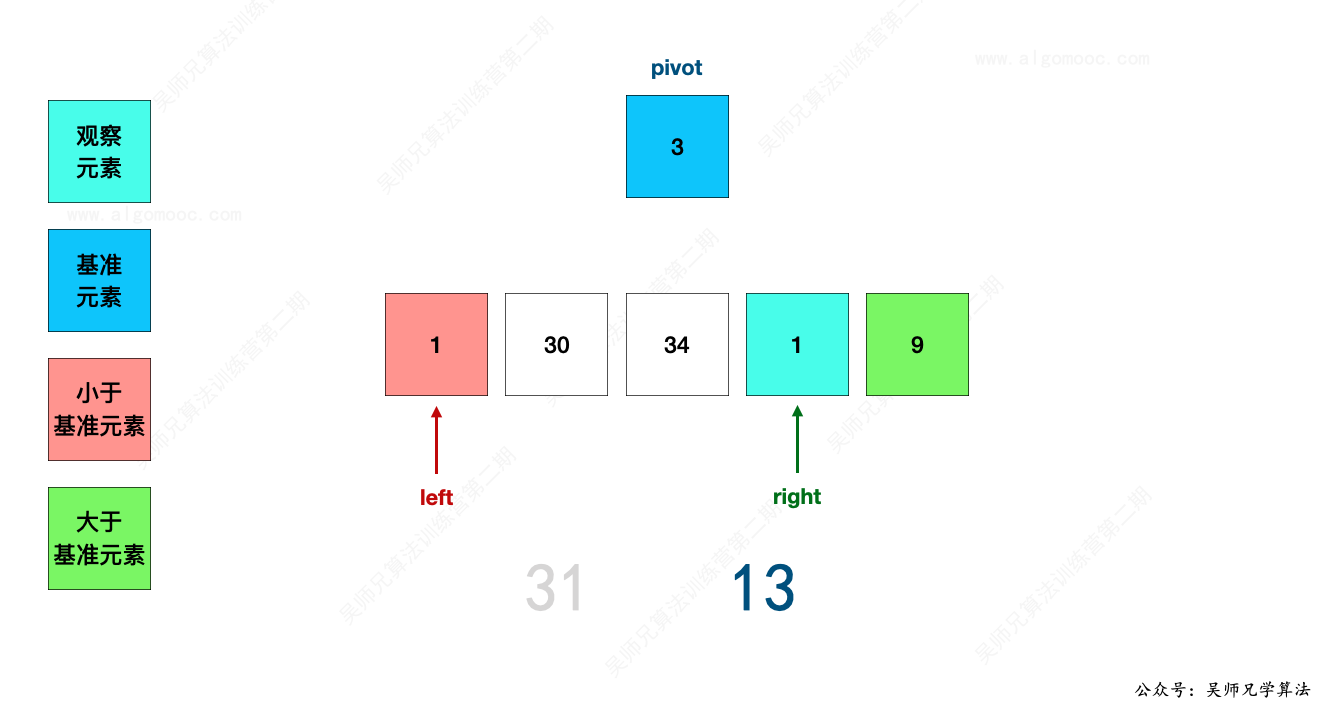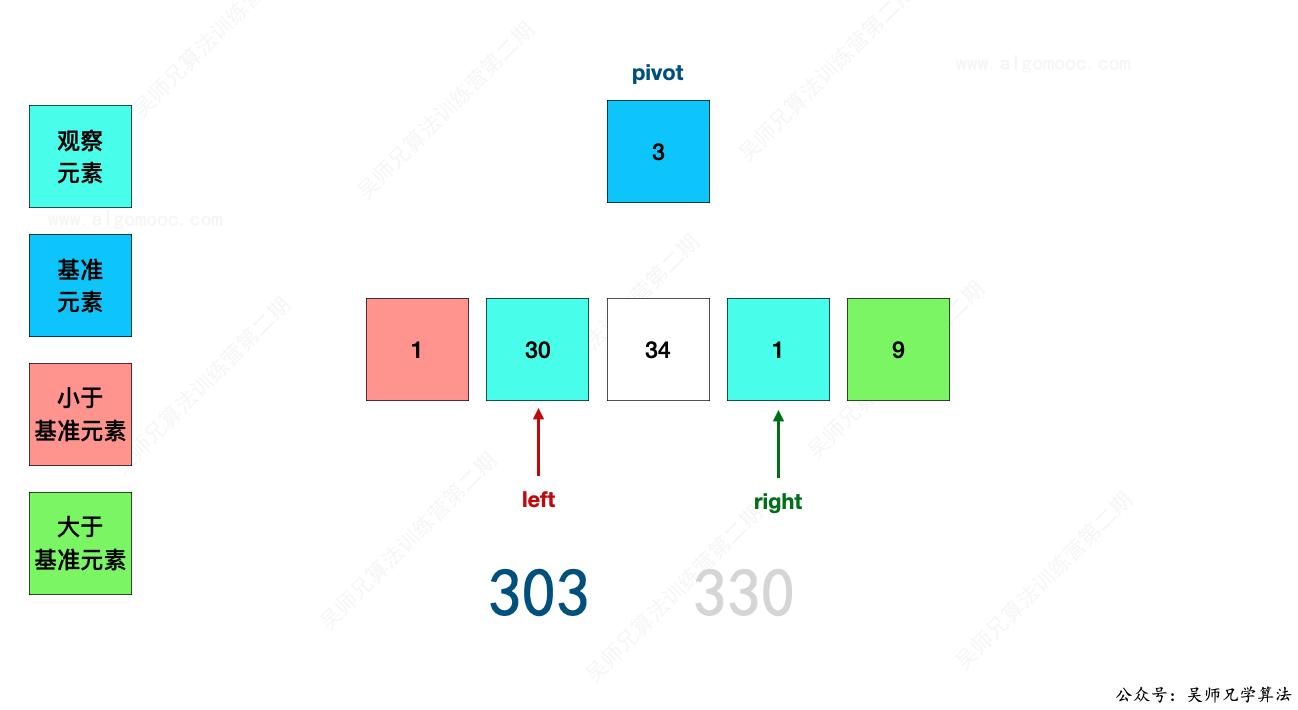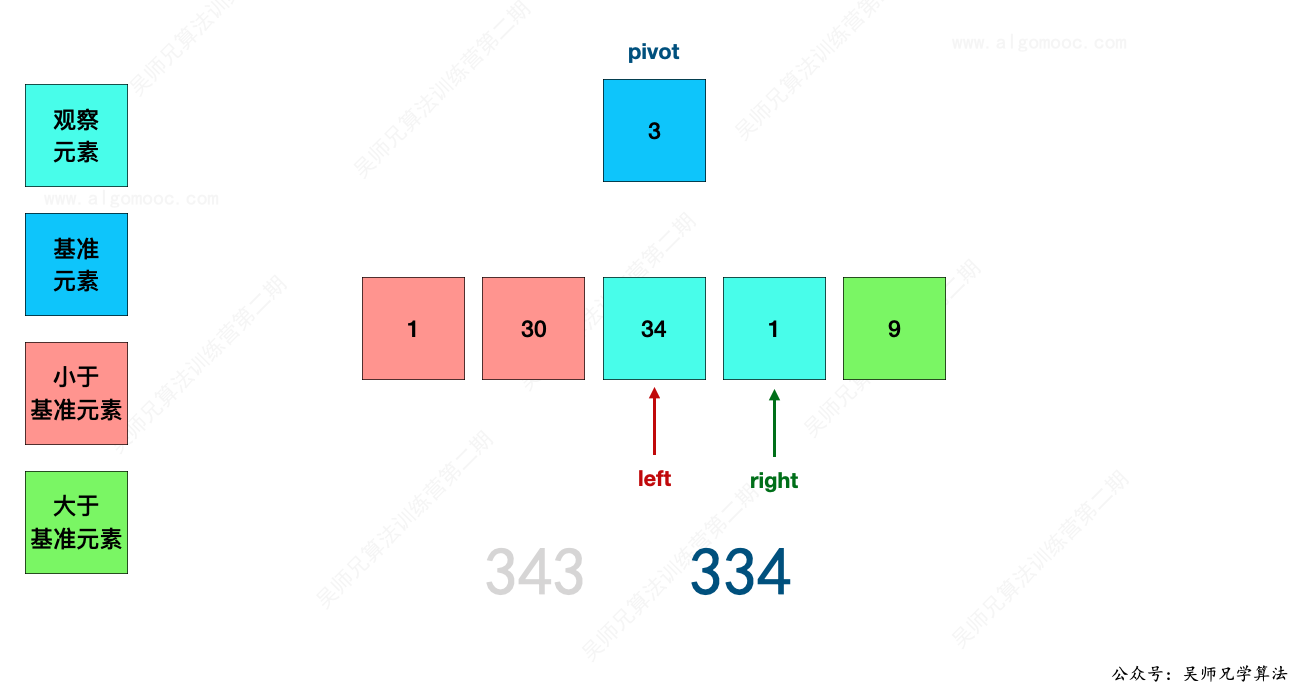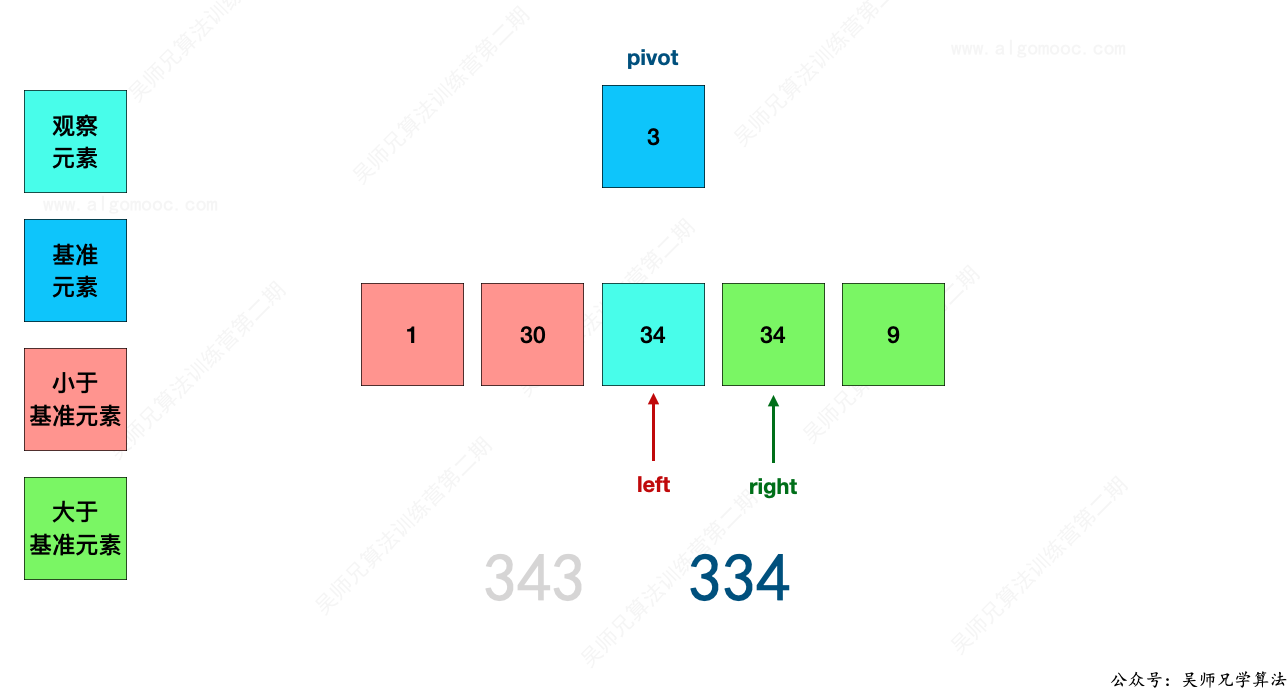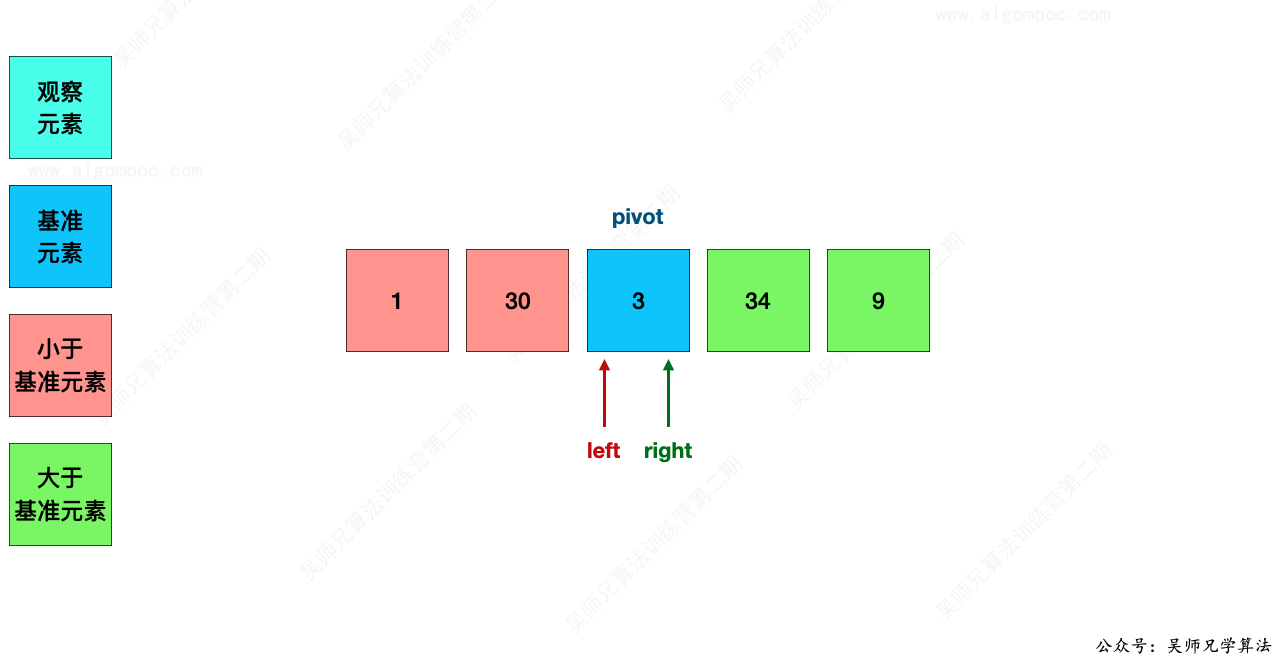1、红色区域的任意数字和蓝色区域的任意数字进行拼接，都是会小于蓝色区域的任意数字和红色区域的任意数字进行拼接。

2、蓝色区域的任意数字和绿色区域的任意数字进行拼接，都是会小于绿色区域的任意数字和蓝色区域的任意数字进行拼接。

## # 三、参考代码

``````// 登录 AlgoMooc 官网获取更多算法图解
// https://www.algomooc.com
// 作者：程序员吴师兄
// 代码有看不懂的地方一定要私聊咨询吴师兄呀
// 剑指 Offer 45. 把数组排成最小的数:https://leetcode-cn.com/problems/ba-shu-zu-pai-cheng-zui-xiao-de-shu-lcof/
class Solution {
public String minNumber(int[] nums) {

// 先将 nums 转换为字符串数组的形式
String[] strs = new String[nums.length];

for(int i = 0; i < nums.length; i++){
strs[i] = String.valueOf(nums[i]);
}
// 通过快速排序的方式，将字符串数组的每个字符按照约定的顺序进行排序
quickSort(strs, 0, strs.length - 1);

// 再把字符串数组转字符串的形式
StringBuilder ans = new StringBuilder();

for(String s : strs)
ans.append(s);

return ans.toString();
}

// 函数传入待排序数组 nums
// 排序区间的左端点 left
// 排序区间的右端点 right
private void quickSort(String[] strs,int left, int right){

// 如果 left 大于等于 right，说明该区间只有 1 个或者没有元素
if( left >= right ){
// 无需再递归划分后再排序，直接返回
return;
}

// 调用函数 partition，将 left 和 right 之间的元素划分为左右两部分
int mid = partition(strs,left,right);

// 划分之后，再对 mid 左侧的元素进行快速排序
quickSort(strs,left,mid - 1);

// 划分之后，再对 mid 右侧的元素进行快速排序
quickSort(strs,mid + 1,right);
}

// 直接套之前的快速排序的代码进行修改
// 原先的小于的含义指的是数值上的小于，比如 1  < 10
// 但现在的小于含义为：a + b 拼凑的字符串小于 b + a 拼凑的字符串
// 比如 a = 1 ，b = 10
// 那么 a + b = “110”，b + a = “101”
// 显然，b + a < a + b
// 也就是说 a 应该放到 b 的后面来拼凑字符串
private int partition(String[] strs, int left ,int right){

// 经典快速排序的写法
// 设置当前区间的第一个元素为基准元素
String pivot = strs[left];

// left 向右移动，right 向左移动，直到 left 和 right 指向同一元素为止
while( left < right ){

// 当 pivot + strs[right] 的字符串小于 strs[right] + pivot 的字符串时
// 说明 strs[right] 在正确的位置上，right 向左移动
while( left < right && (pivot + strs[right]).compareTo(strs[right] + pivot) <= 0 ){
// right 不断的向左移动
right--;
}

// 此时，跳出了上面这个 while 循环，说明 pivot + strs[right] 的字符串大于 strs[right] + pivot 的字符串了
// 说明 strs[right] 不在正确的位置上
// 将此时的 strs[left] 赋值为 strs[right]
// 执行完这个操作，比 pivot 小的这个元素被移动到了左侧
strs[left] = strs[right];

// 当 strs[left] + pivot 的字符串小于 pivot + strs[left] 的字符串时
// 说明 strs[left] 在正确的位置上，left 向右移动
while( left < right && (strs[left] + pivot).compareTo(pivot + strs[left]) <= 0){
// left 不断的向右移动
left++;
}

// 此时，跳出了上面这个 while 循环，说明 strs[left] + pivot 的字符串大于 pivot + strs[left] 的字符串了
// 说明 strs[left] 不在正确的位置上
// 将此时的 strs[right] 赋值为 strs[left]
// 执行完这个操作，比 pivot 大的这个元素被移动到了右侧
strs[right] = strs[left];

}

// 此时，left 和 right 相遇，那么需要将此时的元素设置为 pivot
// 这个时候，pivot 的左侧元素都小于它，右侧元素都大于它
strs[left] = pivot;

// 返回 left
return left;

}
}
``````